# The evolution of DeepLab semantic segmentation system

Last updated on：a month ago

Deep labelling (DeepLab) is one of the most popular tricks to improve semantic segmentation performance. It may be the most accepted method to improve your proposed method performance.

# Introduction

DeepLab has its backbone. Integration makes use of steam convolution, bi-linear interpolation and CRF fine-tuning progress.

## DeepLabv1

DeepLab is a semantic segmentation architecture. First, the input image goes through the network using dilated convolutions. Then the output from the network is bilinearly interpolated and goes through the fully connected CRF to fine-tune the result we obtain the final predictions.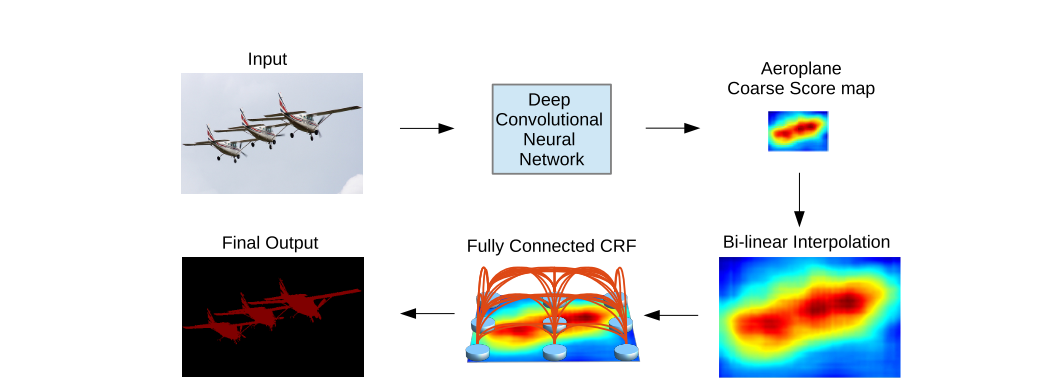The coarse score map from a deep convolutional neural network (with fully convolutional layers) is upsamplled by bi-linear interpolation. A fully connected CRF is applied to refine the segmentation result.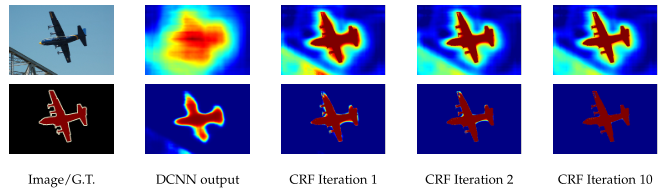Score map (input before softmax function) and belief map (output of softmax function) for Aeroplane. After each mean field iteration, the score (1st row) and belief (2nd row) maps can be generated.

## DeepLabv2

DeepLabv2 is an architecture for semantic segmentation that builds on DeepLab with an atrous spatial pyramid pooling (ASPP) scheme. They have parallel dilated convolutions with different rates applied in the input feature map, then fused. ASPP is used to segment objects at multiple scales robustly.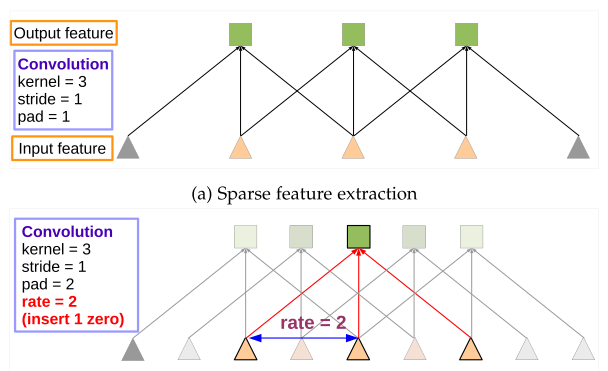## DeepLabv3

DeepLabv3 is a semantic segmentation architecture that improves upon DeepLabv2 with several modifications. To handle the problem of segmenting objects at multiple scales, modules are designed which employ atrous convolution in cascade or in parallel to capture multi-scale contexts by adopting multiple atrous rates.

Furthermore, the ASPP module from DeepLabv2 is augmented with image-level features encoding global context and further boosting performance.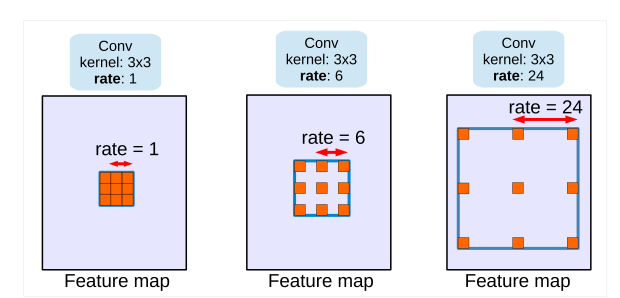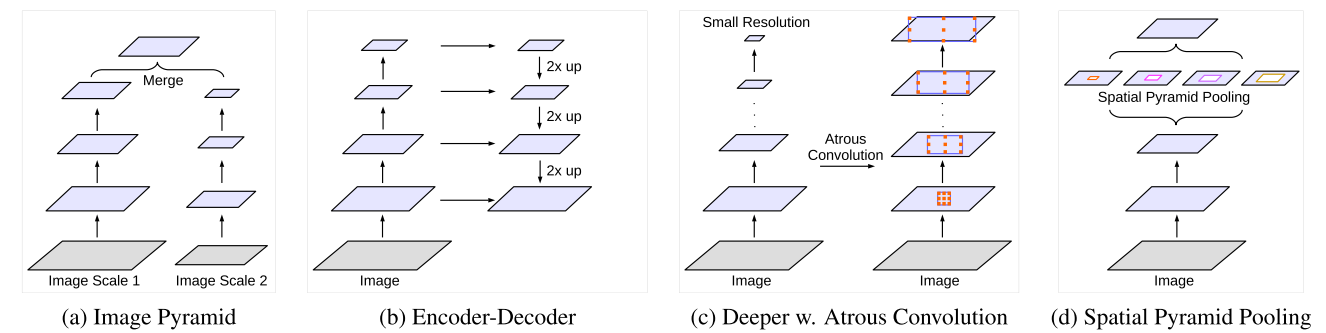## DeepLabv3+

DeepLabv3+ is a semantic segmentation architecture that improves upon DeepLabv3 by adding a simple yet effective decoder module to refine the segmentation results. It removes conditional random field (CRF) and mainly focuses on the spatial pyramid pooling and encoder-decoder structure.

Extends DeepLabv3 by adopting an encoder-decoder structure. The encoder module encodes multi-scale contextual information by applying atrous convolution at multiple scales, while the simple yet effective decoder module refines the segmentation results along object boundaries. The encoder module extract features at an arbitrary resolution by applying atrous convolution.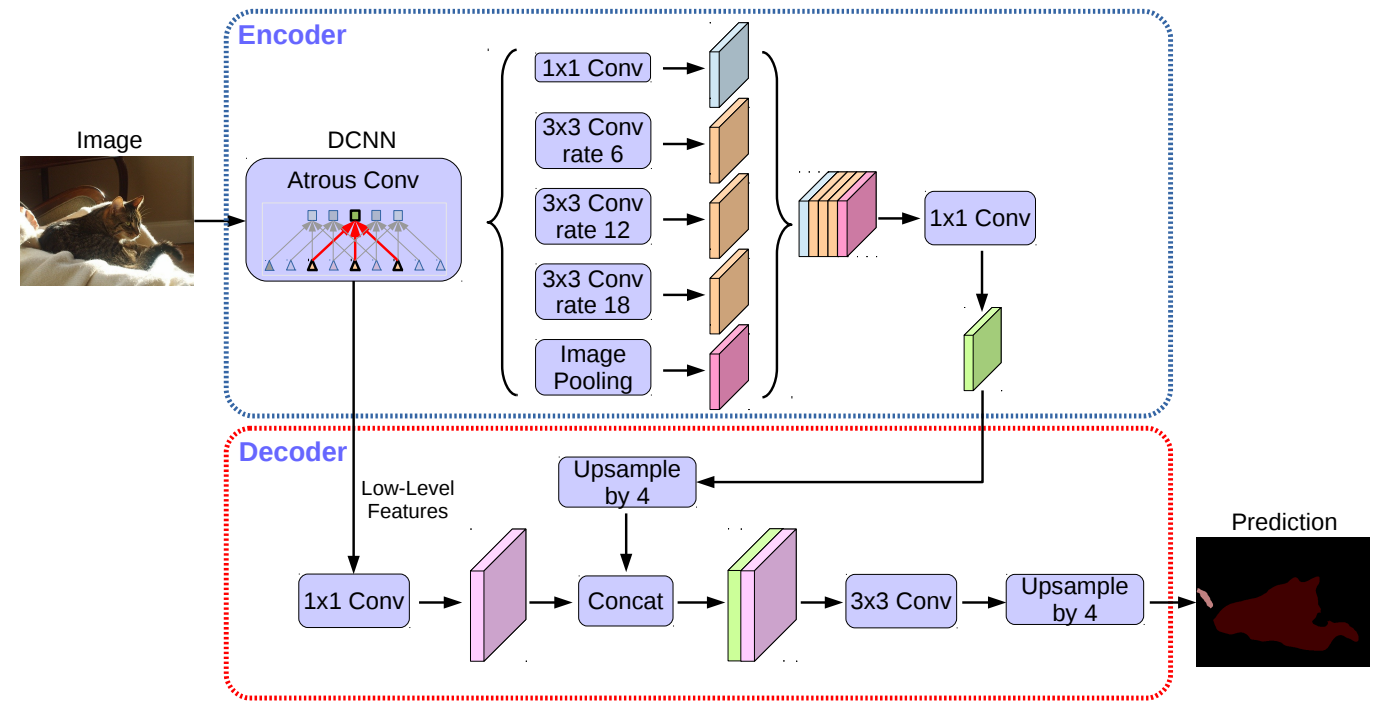Atrous depthwise conv.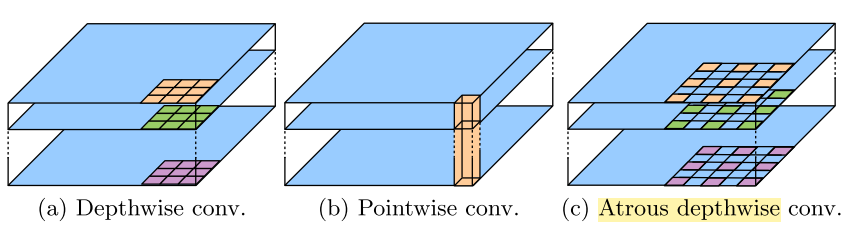DeepLabv3 employs the spatial pyramid pooling module (a) with the encoder-decoder structure (b). The proposed model, DeepLabv3+, contains rich semantic information from the encoder module, while the detailed object boundaries are recovered by the simple yet effective decoder module. (c)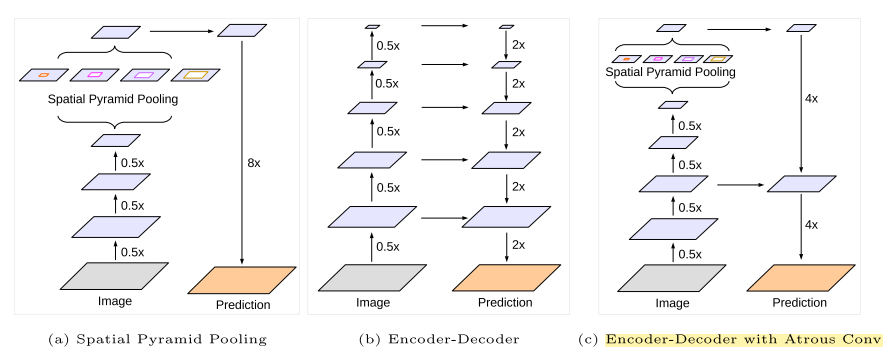DeepLabv3+ is more concise and practical to be integrated into our DCNN.

# Stem convolution

The input and output tensor sizes are the same as the standard convolution. It has a larger kernel size ($7\times 7$).

class _Stem(nn.Sequential):
"""
The 1st conv layer.
Note that the max pooling is different from both MSRA and FAIR ResNet.
"""
def __init__(self, out_ch):
super(_Stem, self).__init__()
self.add_module("conv1", _ConvBnReLU(3, out_ch, 7, 2, 3, 1))
self.add_module("pool", nn.MaxPool2d(3, 2, 1, ceil_mode=True))

# ASPP

The ASPP does not change height and width of the input tensor.

$$H_{\text{out}} = \frac{H _{\text{in}} + 2\times \text{padding}−\text{dilation} \times (\text{kernel size}−1)−1}{\text{stride}}+1$$

$$W_{\text{out}} = \frac{W _{\text{in}} + 2\times \text{padding}−\text{dilation} \times (\text{kernel size}−1)−1}{\text{stride}}+1$$

## DeepLabv2

Sum up all of the output tensors by atrous convolution. To classify the centre pixel (orange), ASPP exploits multi-scale features by employing multiple parallel filters with different rates.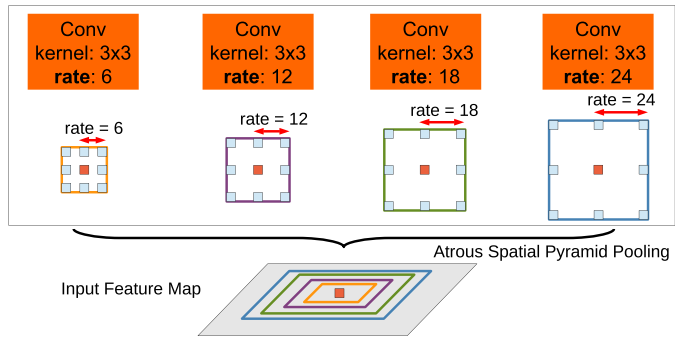Codes:

class _ASPP(nn.Module):
"""
Atrous spatial pyramid pooling (ASPP)
"""
def __init__(self, in_ch, out_ch, rates):
super(_ASPP, self).__init__()
for i, rate in enumerate(rates):
"c{}".format(i),
nn.Conv2d(in_ch, out_ch, 3, 1, padding=rate, dilation=rate, bias=True),
)
for m in self.children():
nn.init.normal_(m.weight, mean=0, std=0.01)
nn.init.constant_(m.bias, 0)
def forward(self, x):
# return sum([stage(x) for stage in self.children()])
for i, stage in enumerate(self.children()):
if i > 0:
Output += stage(x)
else:
Output = stage(x)
return Output

## DeepLabv3

The existence of objects at multiple scales. Alternative architectures are used to capture multi-scale context.

Going deeper with atrous convolution: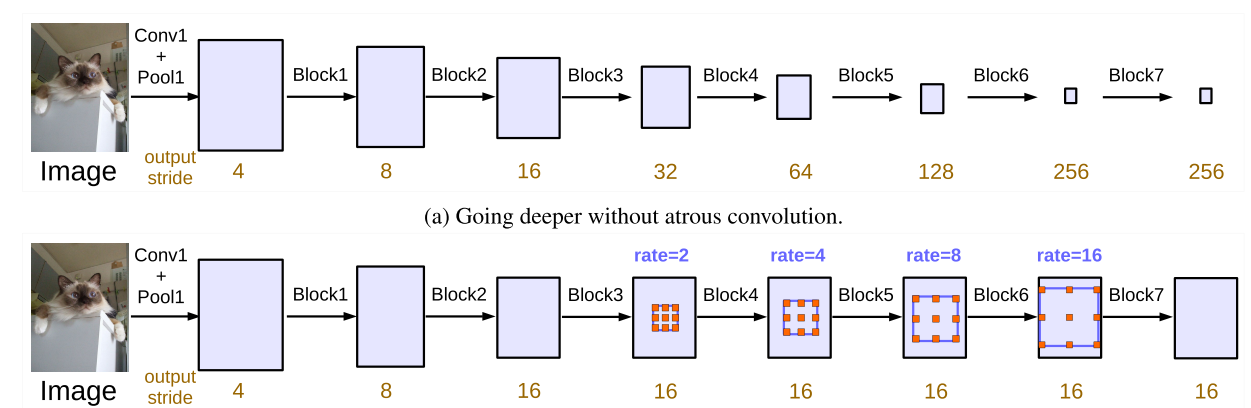As the sampling rate becomes more extensive, the number of valid filter weights (i.e., the weights that are applied to the proper feature region instead of padded zeros) becomes smaller. Parallel modules with atrous convolution (ASPP), augmented with image-level features.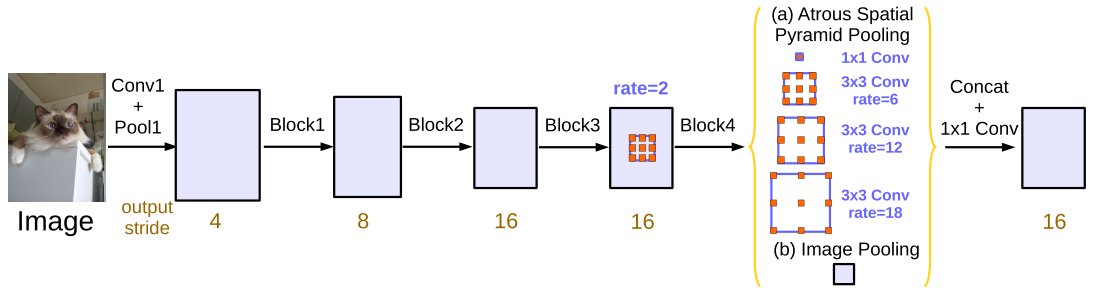DeepLabv3 ASPP, add the image-level feature. In forward(), all tensors are ensembled by torch.cat along channel dimension.

Codes:

class _ASPP(nn.Module):
"""
Atrous spatial pyramid pooling with image-level feature
"""
def __init__(self, in_ch, out_ch, rates):
super(_ASPP, self).__init__()
self.stages = nn.Module()
self.stages.add_module("c0", _ConvBnReLU(in_ch, out_ch, 1, 1, 0, 1))
for i, rate in enumerate(rates):
"c{}".format(i + 1),
_ConvBnReLU(in_ch, out_ch, 3, 1, padding=rate, dilation=rate),
)
def forward(self, x):
return torch.cat([stage(x) for stage in self.stages.children()], dim=1)

## DeepLabv3+

The ASPP module is the same as DeepLabv3.

# Architecture (ResNet example)

## DeepLabv1

Standard convolution is replaced with _Steam, and the block is also changed by different convolution parameters.

Codes:

class DeepLabV1(nn.Sequential):
"""
DeepLab v1: Dilated ResNet + 1x1 Conv
Note that this is just a container for loading the pretrained COCO model and is not mentioned as "v1" in papers.
"""

def __init__(self, n_classes, n_blocks):
super(DeepLabV1, self).__init__()
ch = [64 * 2 ** p for p in range(6)]
self.add_module("layer2", _ResLayer(n_blocks, ch, ch, 1, 1))
self.add_module("layer3", _ResLayer(n_blocks, ch, ch, 2, 1))
self.add_module("layer4", _ResLayer(n_blocks, ch, ch, 1, 2))
self.add_module("layer5", _ResLayer(n_blocks, ch, ch, 1, 4))
self.add_module("fc", nn.Conv2d(2048, n_classes, 1))

## DeepLabv2

Padding = rate, dilation = rate, kernel_size = $3\times 3$.

Codes:

class DeepLabV2(nn.Sequential):
"""
DeepLab v2: Dilated ResNet + ASPP
Output stride is fixed at 8
"""

def __init__(self, n_classes, n_blocks, atrous_rates):
super(DeepLabV2, self).__init__()
ch = [64 * 2 ** p for p in range(6)]
self.add_module("layer2", _ResLayer(n_blocks, ch, ch, 1, 1))
self.add_module("layer3", _ResLayer(n_blocks, ch, ch, 2, 1))
self.add_module("layer4", _ResLayer(n_blocks, ch, ch, 1, 2))
self.add_module("layer5", _ResLayer(n_blocks, ch, ch, 1, 4))

def freeze_bn(self):
for m in self.modules():
if isinstance(m, _ConvBnReLU.BATCH_NORM):
m.eval()

## DeepLabv3

Use multi_grids (different dilation) in layer5, and add FC layers with a Conv 1x1 and a Conv 1x1 to expand channel to NO. classes. dilation=dilation * multi_grids[i],

Codes:

class DeepLabV3(nn.Sequential):
"""
DeepLab v3: Dilated ResNet with multi-grid + improved ASPP
"""
def __init__(self, n_classes, n_blocks, atrous_rates, multi_grids, output_stride):
super(DeepLabV3, self).__init__()
# Stride and dilation
if output_stride == 8:
s = [1, 2, 1, 1]
d = [1, 1, 2, 4]
elif output_stride == 16:
s = [1, 2, 2, 1]
d = [1, 1, 1, 2]
ch = [64 * 2 ** p for p in range(6)]
self.add_module("layer2", _ResLayer(n_blocks, ch, ch, s, d))
self.add_module("layer3", _ResLayer(n_blocks, ch, ch, s, d))
self.add_module("layer4", _ResLayer(n_blocks, ch, ch, s, d))
"layer5", _ResLayer(n_blocks, ch, ch, s, d, multi_grids)
)
concat_ch = 256 * (len(atrous_rates) + 2)
self.add_module("fc1", _ConvBnReLU(concat_ch, 256, 1, 1, 0, 1))
self.add_module("fc2", nn.Conv2d(256, n_classes, kernel_size=1))

## DeepLabv3+

DeepLabv3+ employs a decoder module.

Codes:

class DeepLabV3Plus(nn.Module):
"""
DeepLab v3+: Dilated ResNet with multi-grid + improved ASPP + decoder
"""
def __init__(self, n_classes, n_blocks, atrous_rates, multi_grids, output_stride):
super(DeepLabV3Plus, self).__init__()
# Stride and dilation
if output_stride == 8:
s = [1, 2, 1, 1]
d = [1, 1, 2, 4]
elif output_stride == 16:
s = [1, 2, 2, 1]
d = [1, 1, 1, 2]
# Encoder
ch = [64 * 2 ** p for p in range(6)]
self.layer1 = _Stem(ch)
self.layer2 = _ResLayer(n_blocks, ch, ch, s, d)
self.layer3 = _ResLayer(n_blocks, ch, ch, s, d)
self.layer4 = _ResLayer(n_blocks, ch, ch, s, d)
self.layer5 = _ResLayer(n_blocks, ch, ch, s, d, multi_grids)
self.aspp = _ASPP(ch, 256, atrous_rates)
concat_ch = 256 * (len(atrous_rates) + 2)
self.add_module("fc1", _ConvBnReLU(concat_ch, 256, 1, 1, 0, 1))
# Decoder
self.reduce = _ConvBnReLU(256, 48, 1, 1, 0, 1)
self.fc2 = nn.Sequential(
OrderedDict(
[
("conv1", _ConvBnReLU(304, 256, 3, 1, 1, 1)),
("conv2", _ConvBnReLU(256, 256, 3, 1, 1, 1)),
("conv3", nn.Conv2d(256, n_classes, kernel_size=1)),
]
)
)
def forward(self, x):
h = self.layer1(x)
h = self.layer2(h)
h_ = self.reduce(h)
h = self.layer3(h)
h = self.layer4(h)
h = self.layer5(h)
h = self.aspp(h)
h = self.fc1(h)
h = F.interpolate(h, size=h_.shape[2:], mode="bilinear", align_corners=False)
h = torch.cat((h, h_), dim=1)
h = self.fc2(h)
h = F.interpolate(h, size=x.shape[2:], mode="bilinear", align_corners=False)
return h

# Reference

 Chen, L.C., Papandreou, G., Kokkinos, I., Murphy, K. and Yuille, A.L., 2014. Semantic image segmentation with deep convolutional nets and fully connected crfs. arXiv preprint arXiv:1412.7062.

 Chen, L.C., Papandreou, G., Kokkinos, I., Murphy, K. and Yuille, A.L., 2017. Deeplab: Semantic image segmentation with deep convolutional nets, atrous convolution, and fully connected crfs. IEEE transactions on pattern analysis and machine intelligence, 40(4), pp.834-848.

 Chen, L.C., Papandreou, G., Schroff, F. and Adam, H., 2017. Rethinking atrous convolution for semantic image segmentation. arXiv preprint arXiv:1706.05587.

 Chen, L.C., Zhu, Y., Papandreou, G., Schroff, F. and Adam, H., 2018. Encoder-decoder with atrous separable convolution for semantic image segmentation. In Proceedings of the European conference on computer vision (ECCV) (pp. 801-818).

 tensorflow/models